0%

## 张量的定义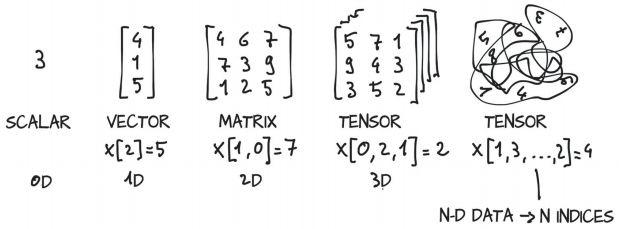## 张量的视图与存储

• 张量，PyTorch中的基本数据结构
• 索引并在PyTorch张量上进行操作以探索和处理数据
• 与NumPy多维数组互操作
• 将计算移至GPU以提高速度

### 张量的视图与存储的定义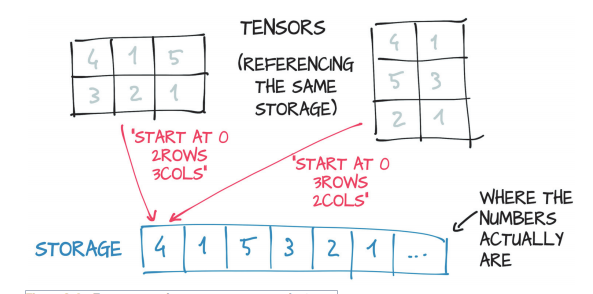### 张量存储的形状（大小）、存储偏移量和步长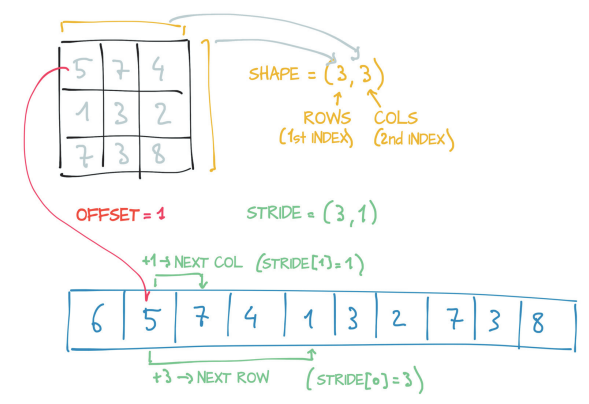### 张量存储对张量操作的影响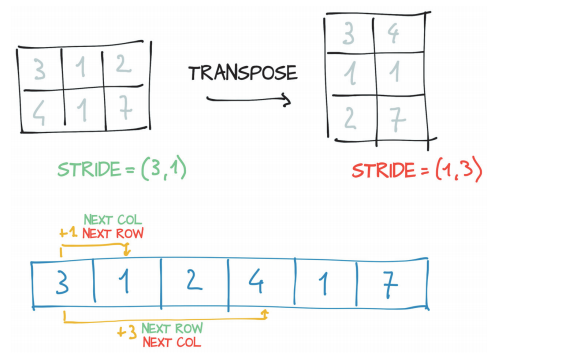### 张量的视图与存储的区别与联系

• 相同存储可以有不同的视图：tensor_B.storage() 与 tensor_B_transpose.storage() 相同，但是 tensor_B 与 tensor_B_transpose 不同。
• 相同的视图可以有不同的存储：tensor_A 与 tensor_B_transpose 相同，但是 tensor_A.storage() 与 tensor_B_transpose.storage() 不同。

## 张量的数据类型和操作

• 数据类型：dtype=int32, float32, string, bool
• 创建张量：tf.convert_to_tensor, tf.constant, tf.zeros, tf.ones, tf.zeros_like, tf.fill, tf.random.normal, tf.random.uniform, tf.range
• 索引与切片：A, A[1, 2, 1], A[ :, :, 0:3:2], A[..., 0:3:2]
• 维度变换：tf.reshape, tf.expand_dims, tf.squeeze, tf.transpose
• 数据复制：tf.tile
• 数学运算：+, -, *, /, //, %, **, tf.pow, tf.square, tf.sqrt, tf.math.log, tf.matmul, @
• 合并与分割：tf.concat, tf.stack, tf.split, tf.unstack
• 统计量：tf.norm, tf.reduce_max min mean sum, tf.argmax, tf.argmin
• 张量比较：tf.equal
• 填充与复制：tf.pad, tf.keras.preprocessing.sequence.pad_sequences, tf.tile
• 数据限幅：tf.maximum, tf.minimum, tf.clip_by_value
• 数据收集：tf.gather, tf.gather_nd
• 掩码：tf.boolean_mask
• 条件：tf.where
• 数据刷新：tf.scatter_nd
• 采样：tf.meshgrid

TODO：增加PyTorch版本张量的操作

### 数值类型

1. 标量，0维张量，主要用于模型损失和各种测量指标的表示，比如准确率、精度等；
2. 向量，1维张量，主要用于表示模型权重中的偏置b；
3. 矩阵，2维张量，主要用于表示线性模型Dense中的权重矩阵W；
4. 张量，3维张量主要用于序列数据，它的格式是 [batch_size, sequence length, feature length]，比如自然语言处理中句子的嵌入表示 [批量大小，句子长度，词嵌入维度]。4维张量主要用于图像数据，它的格式是 [batch_size, length, width, channel]。更高维度张量较少使用。

## 张量操作的意义

### 神经网络为什么要使用使用偏置b？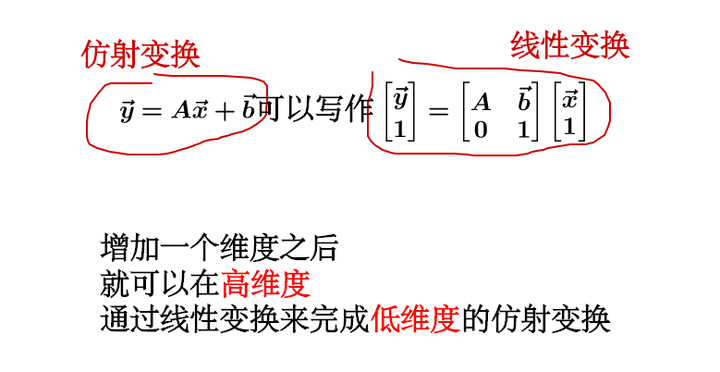### 内积（点积、数量积）

$a \cdot b = a_1b_1 + a_2b_2 + … + a_nb_n$。

$a \cdot b = (a^T) * b$，这里的$a^T$指示矩阵a的转置。

### 外积（向量积）

$(x_1,y_1,z_1) \times (x_2,y_2,z_2)=(y_1z_2-y_2z_1,z_1x_2-z_2x_1,x_1y_2-x_2y_1)$

## 张量与现实数据的转换

• 将不同类型的实际数据表示为PyTorch张量
• 处理各种数据类型，包括电子表格、时间序列、文本、图像、医疗成像、视频、音频
• 从文件中加载数据
• 将数据转换为张量
• 对张量进行整形（shaping），使其可以用作神经网络模型的输入